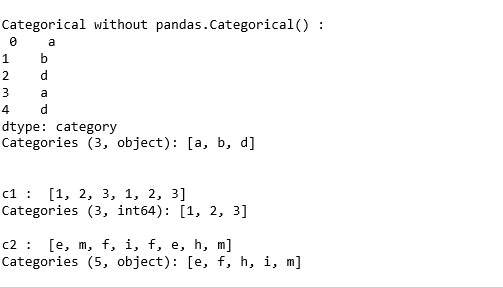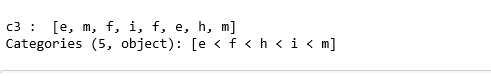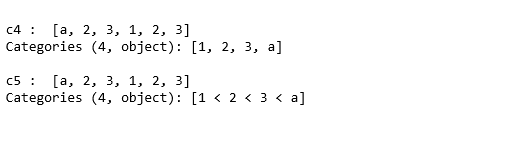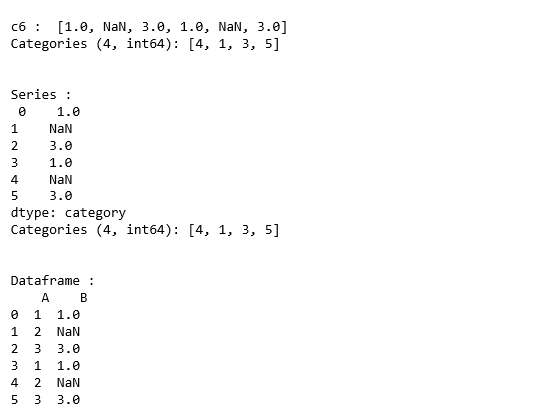# Python | Pandas.Categorical()

pandas.Categorical(val, categories = None, ordered = None, dtype = None) : It represents a categorical variable. Categoricals are a pandas data type that corresponds to the categorical variables in statistics. Such variables take on a fixed and limited number of possible values. For examples – grades, gender, blood group type etc.
Also, in the case of categorical variables, logical order is not the same as categorical data e.g. “one”, “two”, “three”. But the sorting of these variables uses logical order.

````Parameters- `val        : [list-like] The values of categorical.
categories : [index like] Unique categorisation of the categories.
ordered    : [boolean] If false, then the categorical is treated as unordered.
dtype      : [CategoricalDtype] an instance.

`Error- `ValueError :  If the categories do not validate.
TypeError  :  If an explicit ordered = True but categorical can't be sorted.

Return- Categorical varibale```

Code:

 `# Python code explaining  ` `# numpy.pandas.Categorical() ` ` `  `# importing libraries ` `import` `numpy as np ` `import` `pandas as pd ` ` `  `# Categorical using dtype ` `c ``=` `pd.Series([``"a"``, ``"b"``, ``"d"``, ``"a"``, ``"d"``], dtype ``=``"category"``) ` `print` `(``"\nCategorical without pandas.Categorical() : \n"``, c) ` ` `  ` `  `c1 ``=` `pd.Categorical([``1``, ``2``, ``3``, ``1``, ``2``, ``3``]) ` `print` `(``"\n\nc1 : "``, c1) ` ` `  `c2 ``=` `pd.Categorical([``'e'``, ``'m'``, ``'f'``, ``'i'``, ` `                     ``'f'``, ``'e'``, ``'h'``, ``'m'` `]) ` `print` `(``"\nc2 : "``, c2) `

Output :`# Ordered = True ` `c3 ``=` `pd.Categorical([``'e'``, ``'m'``, ``'f'``, ``'i'``, ` `                     ``'f'``, ``'e'``, ``'h'``, ``'m'` `], ordered ``=` `True``) ` `print` `(``"\nc3 : "``, c3) `

Output :`# Mixed categories ` `c4 ``=` `pd.Categorical([``'a'``, ``2``, ``3``, ``1``, ``2``, ``3``]) ` `print` `(``"\nc4 : "``, c4) ` ` `  `c5 ``=` `pd.Categorical([``'a'``, ``2``, ``3``, ``1``, ``2``, ``3``], ordered ``=` `True``) ` `print` `(``"\nc5 : "``, c5) `

Output :`# using categories attribute ` `c6 ``=` `pd.Categorical([``1``, ``2``, ``3``, ``1``, ``2``, ``3``], categories ``=` `[``4``, ``1``, ``3``, ``5``]) ` `print` `(``"\nc6 : "``, c6) ` ` `  `print``(``"\n\nSeries : \n"``, pd.Series(c6)) ` ` `  `df ``=` `pd.DataFrame({``"A"``:[``1``, ``2``, ``3``, ``1``, ``2``, ``3``]}) ` `df[``"B"``] ``=` `c6 ` `print` `(``"\n\nDataframe : \n"``, df) `

Output :My Personal Notes arrow_drop_upIf you like GeeksforGeeks and would like to contribute, you can also write an article using contribute.geeksforgeeks.org or mail your article to contribute@geeksforgeeks.org. See your article appearing on the GeeksforGeeks main page and help other Geeks.

Please Improve this article if you find anything incorrect by clicking on the "Improve Article" button below.

Article Tags :

Be the First to upvote.

Please write to us at contribute@geeksforgeeks.org to report any issue with the above content.